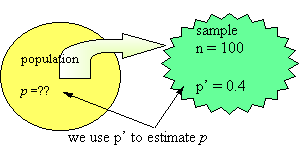### CFA Practice Question

There are 985 practice questions for this topic.

### CFA Practice Question

For a 90% confidence interval for the population proportion, p, if the sample proportion, p', is 0.4 and the sample size is n = 100 then the error term, E, is ______ (to the nearest 0.001).A. 0.049
B. 0.004
C. 0.081

The error term is some number of standard deviations, in this case, 1.645 (the cutoff for the top 5% of the normal distribution). The standard deviation of the sampling distribution is sqrt[(0.4)(1 - 0.4)/100] = 0.049. Now, E = 1.64(0.049) = 0.081. Thus, the confidence interval for p is 0.4 - 0.081 < p < 0.4 + 0.081.User Comment
danlan How to get sqrt(0.4*0.6/100)?
akanimo am lost here ... can anyone explain how variance = s^2 = p'(1 - p')

i havent seen this formula anywhere yet???
sunilcfa its done with binomial dis
surob Didn't understand. In binomial, variance is equal to np(1-p), not p(1-P). Can someone clarify?
weiwei see the http://mathworld.wolfram.com/SampleProportion.html
Tomcat82 I get it. The formula for var of binomial is var=np(1-p)

The formula for see=sd/(n^(1/2)), so var is this squared. since you have n in the numerator, and n^2 in the demoninator, they cancel out, so you're just left with n in the demoninator, and no n in the numerator. Now remember, this is the formula for the variance. to get the SEE, you have to take the sqare root, thus you're left with the equation from above
StanleyMo Normal Approximations for Counts and Proportions
For large values of n, the distributions of the count X and the sample proportion are approximately normal. This result follows from the Central Limit Theorem. The mean and variance for the approximately normal distribution of X are np and np(1-p), identical to the mean and variance of the binomial(n,p) distribution. Similarly, the mean and variance for the approximately normal distribution of the sample proportion are p and (p(1-p)/n).
cfabuzz simply, variance of distribution mean = s^2/n
the variance(s^2)in binomial is np(1-p)
so we get np(1-p)/n --> n cancel out and we get var = p(1-p), so standard deviation(s)is square root of p(1-p)
E = 1.645* s/ square root of n
E = 1.645* [p(1-p)/n]^1/2
panvino Good question!
johntan1979 http://www.stat.yale.edu/Courses/1997-98/101/binom.htm

pop mean, var = np, np(1-p)
sample mean, var = p, [p(1-p)]/n

Too easy to assume sample var is divided by only one n to become p(1-p) like the mean.
sgossett86 I had to kinda backtrack to learn this one..
We're talking about a proportion, so assume that the Single Bernoulli Variable rules apply. They were one of the important formulas we went over regarding binomials. Review it if necessary.

Variance=p(1-p)=.4(1-.4)

sd=(.4(1-.4))^.5=.48990

We're solving for E

E=Z(a/2)*(sd/N^.5)=(1.645)*(.48990/100^.5)=.08059
sgossett86 I couldn't got this without initial guidance from all your suggestions above, but that's the formula breakdown.
lndcobra What makes you realise that this is talking about a Binomial Distribution, and hence arriving at

var =s^2 = np(1-p)
lawlee I still don't get how the n in the numerator is offset, squarting n^1/2 on the denominator get you to n, not n^2.
Can someone clarify?